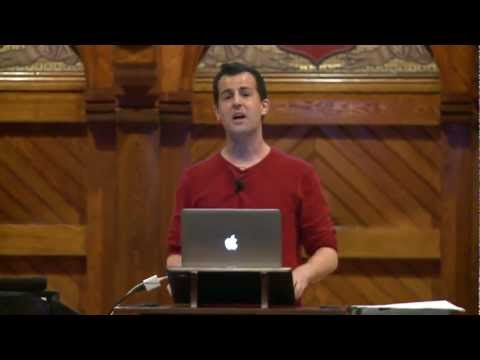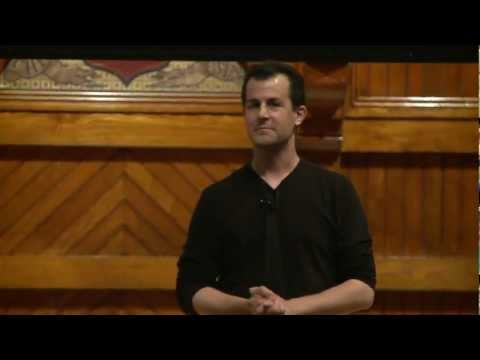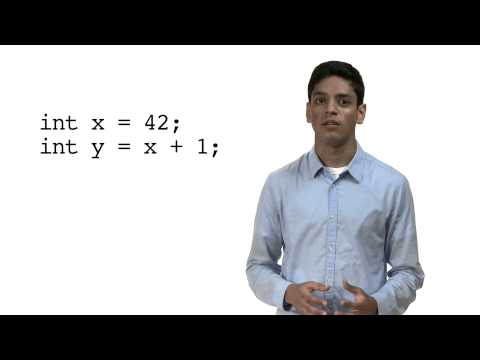# Math

## Notes

The math blocks you've used in Scratch can all be recreated in C!

Numerical data in C is classified into several different types. From a variable’s type, you’ll be able to tell what kind of data it can store, how much data it can store, and which operations can be performed on this data.

• An int is a whole number. The appliance uses 32 bits to store an int.
• A float is a number with a decimal point. The appliance uses 32 bits to store a float.
• A double is a number with a decimal point, but with more space for precision after the decimal point. The appliance uses 64 bits to store a double.
• A long long is a whole number that is twice as big in memory as an int. The appliance uses 64 bits to store a long long.

Here, we sum two variables of type int. Let's think very carefully about what's happening as these three lines of code execute:

• The first line declares an int named x. More precisely, it asks the operating system for enough memory to store an int and gives that chunk of memory the name x.
• The second line initializes x by assigning it a value of 2. Remember that in C, a single equal sign is called an assignment operator. Whatever's to the right of the equal sign will be stored in the variable to the left of the equal sign.
• The third line declares and initializes an int named y in a single line of code.

Let’s try some division next, and store the result of our division in a float because we know it will be a decimal value.

%.2f tells the OS to print a floating-point number, but only to two decimal places.

What will print?

0.00

Why?

1 and 10 are ints. In C, an int divided by an int is an int and any decimal places will be truncated off.

We can fix this!

We can use this syntax (desired data type within parentheses) to cast the ints 1 and 10 to floats. This is called typecasting.

A float divided by a float is a float, which preserves decimal places! What will print this time? 0.10

Another way to accomplish the same thing is to replace the ints 1 and 10 with floats 1.0 and 10.0.

This method also results in the printing of 0.10.

Remember "order of operations" from elementary school? The same concept applies in programming and is called operator precedence.

1. 27

2. 22

3. 10

The modulo operator gives you the remainder of the division of the first number by the second.

1. 5

2. 3

3. 0

4. 1

What will be printed out?

2

Why is it that 0.10000000149011611938 will print rather than simply 0.10000000000000000000?

Some fractions like 0.1 can't be represented precisely using a finite number of binary bits. This is known as floating point imprecision and can cause significant rounding errors if you're not careful!## Slides ( / )## Temperature Conversion

Prerequisites:

Write a program that prompts the user to enter a temperature in Fahrenheit, converts the temperature to Celsius, and prints out the result with one decimal place.

``````jharvard@run.cs50.net (~): ./a.out
Temperature in F: 100
Temperature in C: 37.8``````

Try out some pseudocode here!
```#include <cs50.h>
#include <stdio.h>

int main(int argc, string argv[])
{

// TODO: prompt user for temp in F, convert to C, print result

}
```

## Percentages

Prerequisites:

Write a program that prompts the user for a non-negative numerator and a positive denominator and prints the fraction as a percent to two decimal places.

``````jharvard@run.cs50.net (~): ./a.out
non-negative numerator: 1
positive denominator: 2
50.00%``````

Try out some pseudocode here!
```#include <cs50.h>
#include <stdio.h>

int main(int argc, string argv[])
{

// TODO: prompt user for fraction, convert to %, print %

}
```

## Videos#### Monday, Week 1

Math in C#### Wednesday, Week 1

More math in C#### Vipul's Variables Short

Manipulating variables